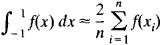# Chebyshev Formula

The following article is from The Great Soviet Encyclopedia (1979). It might be outdated or ideologically biased.

## Chebyshev Formula

a formula for the approximate computation of a definite integral:The formula is accurate for polynomials of degree not greater than n – 1, where n is the number of nodes. The values of the xi have been computed for some n. In the case of n = 9, for example, x1 = –x9 = 0.911589, x2 = –x8 = 0.601019, x3 = –x7 = 0.528762, x4 = –x6 = 0.167906, and x5 = 0. For n = 8 and n > 9, the xi have complex values; the Chebyshev formula can therefore be used only for n = 1, 2, 3, 4, 5, 6, 7, and 9. The for mula was derived by P. L. Chebyshev in 1873.

The Great Soviet Encyclopedia, 3rd Edition (1970-1979). © 2010 The Gale Group, Inc. All rights reserved.
Mentioned in ?
References in periodicals archive ?
The initial mechanism (links 0, 1) together with the fourth class second order structural group, which consists of a set of four links 2.5 (n=5) along with six kinematic pairs of the fifth class A, B, C, D, E, K ([p.sub.5]=6) form a fourth class mechanism with a degree of freeness equal to 1 according to the Chebyshev formula: W=3n-2[p.sub.5]-[p.sub.4], that is form a mechanism with one driving crank.
7 (n = 6) with nine kinematic pairs of 5th class--A, B, C, D, E, L, K, M, N ([p.sub.5] = 9) - forming the mechanism of the 4th class with degree of freedom 1 by Chebyshev formula (mechanism with a driving crank):
(1) This formula is sometimes called Chebyshev formula, since in the one-dimensional case it is based on the expansion of a function in terms of Chebyshev polynomials [T.sub.i] of the first kind.

Site: Follow: Share:
Open / Close